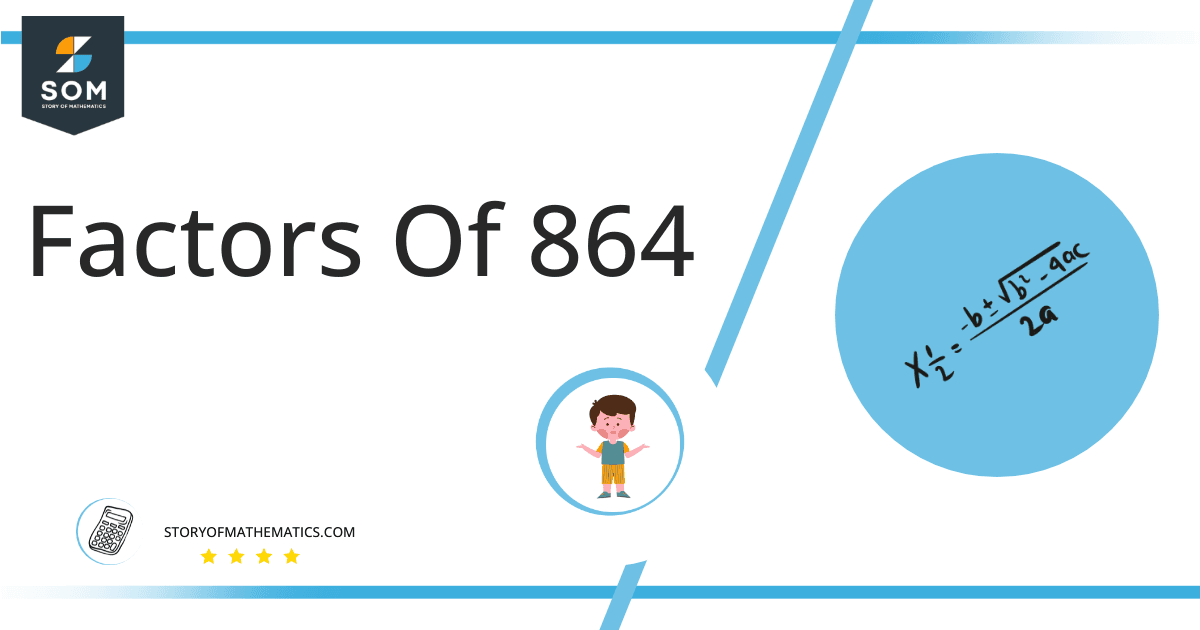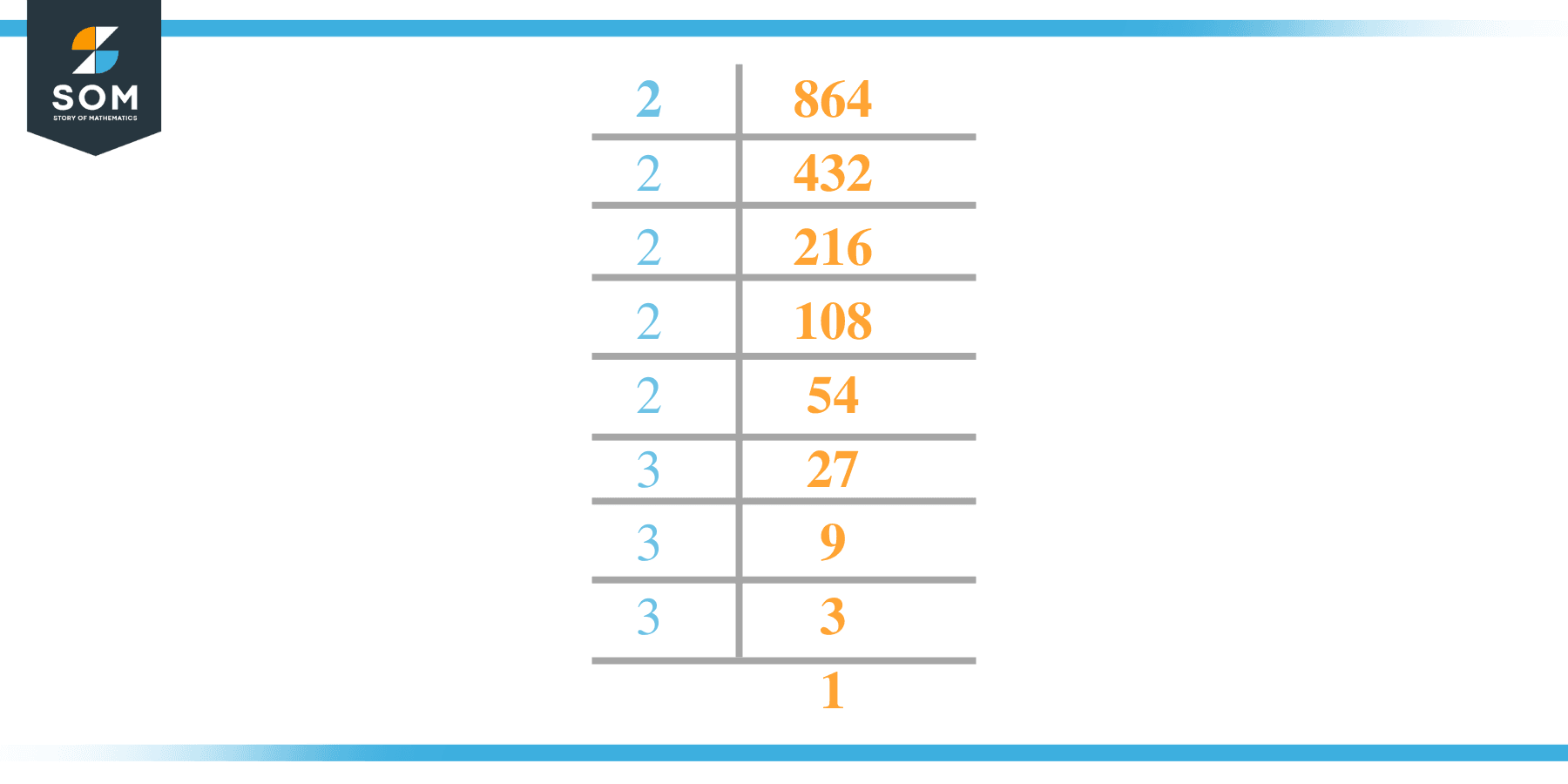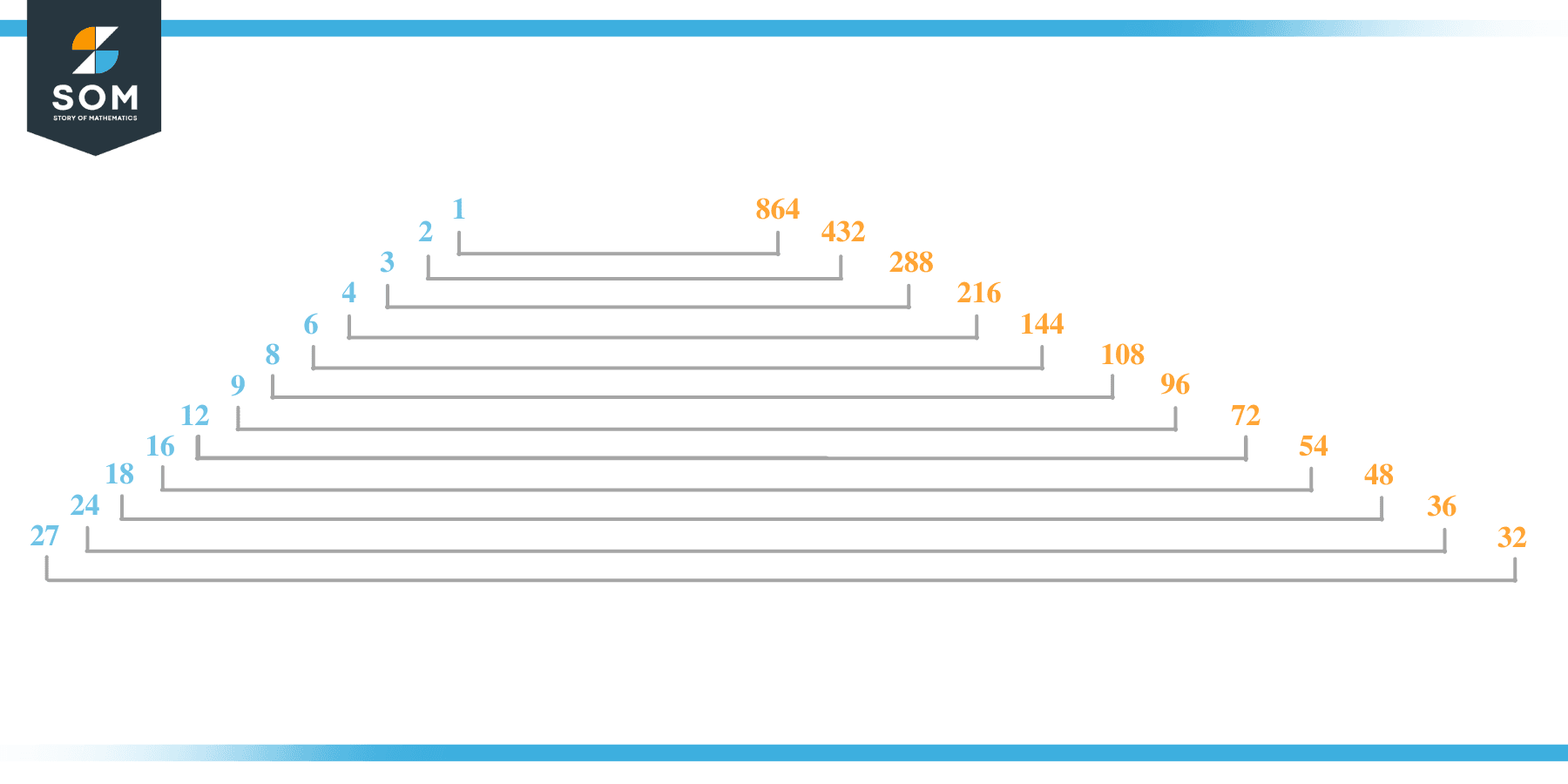# Factors of 864: Prime Factorization, Methods, and Examples

These numbers which are about 24 are 1, 2, 3, 4, 6, 8, 9, 12, 16, 18, 24, 27, 32, 36, 48, 54, 72, 96, 108, 144, 216, 288, 432, and 864 we said them factors of 864  because these are evenly divisible by 864 with give no residue left.### Factors of 864

Here are the factors of number 864.

Factors of 864: 1, 2, 3, 4, 6, 8, 9, 12, 16, 18, 24, 27, 32, 36, 48, 54, 72, 96, 108, 144, 216, 288, 432, and 864

### Negative Factors of 864

The negative factors of 864 are similar to its positive aspects, just with a negative sign.

Negative Factors of 864: -1, -2, -3, -4, -6, -8, -9, -12, -16, -18, -24, -27, -32, -36, -48, -54, -72, -96, -108, -144, -216, -288, -432, and -864

### Prime Factorization of 864

The prime factorization of 864 is the way of expressing its prime factors in the product form.

Prime Factorization: 2 x 2 x 2 x 2 x 2 x 3 x 3 x 3

In this article, we will learn about the factors of 864 and how to find them using various techniques such as upside-down division, prime factorization, and factor tree.

## What Are the Factors of 864?

The factors of 864 are 1, 2, 3, 4, 6, 8, 9, 12, 16, 18, 24, 27, 32, 36, 48, 54, 72, 96, 108, 144, 216, 288, 432, and 864. These numbers are the factors as they do not leave any remainder when divided by 864.

The factors of 864 are classified as prime numbers and composite numbers. The prime factors of the number 864 can be determined using the prime factorization technique.

## How To Find the Factors of 864?

You can find the factors of 864 by using the rules of divisibility. The divisibility rule states that any number, when divided by any other natural number, is said to be divisible by the number if the quotient is the whole number and the resulting remainder is zero.

To find the factors of 864, create a list containing the numbers that are exactly divisible by 864 with zero remainders. One important thing to note is that 1 and 864 are the 864’s factors as every natural number has 1 and the number itself as its factor.

1 is also called the universal factor of every number. The factors of 864 are determined as follows:

$\dfrac{864}{1} = 864$

$\dfrac{864}{2} = 432$

$\dfrac{864}{3} = 288$

$\dfrac{864}{4} = 216$

$\dfrac{864}{6} = 144$

$\dfrac{864}{8} = 108$

$\dfrac{864}{9} = 96$

$\dfrac{864}{12} = 72$

$\dfrac{864}{16} = 54$

$\dfrac{864}{18} = 48$

$\dfrac{864}{24} = 36$

$\dfrac{864}{27} = 32$

$\dfrac{864}{864} = 1$

Therefore, 1, 2, 3, 4, 6, 8, 9, 12, 16, 18, 24, 27, 32, 36, 48, 54, 72, 96, 108, 144, 216, 288, 432, and 864 are the factors of 864.

### Total Number of Factors of 864

For 864, there are 24 positive factors and 24 negative ones. So in total, there are 48 factors of 864.

To find the total number of factors of the given number, follow the procedure mentioned below:

1. Find the factorization/prime factorization of the given number.
2. Demonstrate the prime factorization of the number in the form of exponent form.
3. Add 1 to each of the exponents of the prime factor.
4. Now, multiply the resulting exponents together. This obtained product is equivalent to the total number of factors of the given number.

By following this procedure, the total number of factors of 864 is given as:

Factorization of 864 is 2 x 2 x 2 x 2 x 2 x 3 x 3 x 3

The exponent of 2 and 3 is 5,3.

Adding 1 to each and multiplying them together results in 24.

Therefore, the total number of factors of 864 is 48. Twenty-four are positive, and twenty-four factors are negative.

### Important Notes

Here are some essential points that must be considered while finding the factors of any given number:

• The factor of any given number must be a whole number.
• The factors of the number cannot be in the form of decimals or fractions.
• Factors can be positive as well as negative.
• Negative factors are the additive inverse of the positive factors of a given number.
• The factor of a number cannot be greater than that number.
• Every even number has 2 as its prime factor, the smallest prime factor.

## Factors of 864 by Prime FactorizationThe number 864 is a composite. Prime factorization is a valuable technique for finding the number’s prime factors and expressing the number as the product of its prime factors.

Before finding the factors of 864 using prime factorization, let us find out what prime factors are. Prime factors are the factors of any given number that are only divisible by 1 and themselves.

To start the prime factorization of 864, start dividing by its most minor prime factor. First, determine that the given number is either even or odd. If it is an even number, then 2 will be the smallest prime factor.

Continue splitting the quotient obtained until 1 is received as the quotient. The prime factorization of 864 can be expressed as:

864 = 2 x 2 x 2 x 2 x 2 x 3 x 3 x 3

## Factors of 864 in PairsThe factor pairs are the duplet of numbers that, when multiplied together, result in the factorized number. Factor pairs can be more than one depending on the total number of factors given.

For 864, the factor pairs can be found as:

1 x 864 = 864

2 x 432 = 864

3 x 288 = 864

4 x 216 = 864

6 x 144 = 864

8 x 108 = 864

9 x 96 = 864

12 x 72 = 864

16 x 54 = 864

18 x 48 = 864

24 x 36 = 864

27 x 32 = 864

The possible factor pairs of 864 are given as (1, 864), (2, 432), (3, 288), (4, 216), (6, 144), (8, 108), (9, 96), (12, 72), (16, 54), (18, 48), (24, 36), and (27, 32).

All these numbers in pairs, when multiplied, give 864 as the product.

The negative factor pairs of 864 are given as:

-1 x -864 = 864

-2 x -432 = 864

-3 x -288 = 864

-4 x -216 = 864

-6 x -144 = 864

-8 x -108 = 864

-9 x -96 = 864

-12 x -72 = 864

-16 x -54 = 864

-18 x -48 = 864

-24 x -36 = 864

-27 x -32 = 864

It is important to note that in negative factor pairs, the minus sign has been multiplied by the minus sign, due to which the resulting product is the original positive number. Therefore, -1, -2, -3, -4, -6, -8, -9, -12, -16, -18, -24, -27, -32, -36, -48, -54, -72, -96, -108, -144, -216, -288, -432, and -864 are called negative factors of 864.

The list of all the factors of 864, including positive as well as negative numbers, is given below.

Factor list of 864: 1, -1, 2, -2, 3, -3, 4, -4, 6, -6, 8, -8, 9, -9, 12, -12, 16, -16, 18, -18, 24, -24, 27, -27, 32, -32, 36, -36, 48, -48, 54, -54, 72, -72, 96, -96, 108, -108, 144, -144, 216, -216, 288, -288, 432, -432, 864 and -864

## Factors of 864 Solved Examples

To better understand the concept of factors, let’s solve some examples.

### Example 1

How many factors of 864 are there?

### Solution

The total number of Factors of 864 is 24.

Factors of 864 are 1, 2, 3, 4, 6, 8, 9, 12, 16, 18, 24, 27, 32, 36, 48, 54, 72, 96, 108, 144, 216, 288, 432, and 864.

### Example 2

Find the factors of 864 using prime factorization.

### Solution

The prime factorization of 864 is given as:

864 $\div$ 2 = 432

432 $\div$ 2 = 216

216 $\div$ 2 = 108

108 $\div$ 2 = 54

54 $\div$ 2 = 27

27 $\div$ 3 = 9

9 $\div$ 3 = 3

3 $\div$ 3 = 1

So the prime factorization of 864 can be written as:

2 × 2 × 2 × 2 × 2 × 3 × 3 × 3 = 864